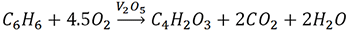MORE IN Applied Chemistry 2
MU First Year Engineering (Semester 2)
Applied Chemistry 2
December 2012
Total marks: --
Total time: --
INSTRUCTIONS
(1) Assume appropriate data and state your reasons
(2) Marks are given to the right of every question
(3) Draw neat diagrams wherever necessary

Answer any five from the following:
1 (a) Give the characteristics of a good fuel.
3 M
1 (b) Give the composition, properties and uses of Gun metal.
3 M
1 (c) What is Green Chemistry? Give its significance.
3 M
1 (d) What are composite materials? What are the applications of composites?
3 M
1 (e) Explain homogenous and heterogenous catalysis.
3 M
1 (f) Explain Galvanic corrosion.
3 M
1 (g) In a Kjeldahl's apparatus, 3.5g of the coal sample was analysed. The NH3 gas evolved was absorbed in 50ml of 0.1N H2SO4, The residual H2SO4 required 15ml of 0.1N NaOH for neutralisation. Calculate percentage of nitrogen in the sample.
3 M

2 (a) Calculate the weight and volume of air required for complete combustion of 1kg of coal containing; C = 65%, H = 4%, O = 7%, N = 3%, moisture = 15% and the remaining is ash. (molecular weight of air = 28.94g)
6 M
2 (b) Define corrosion. Explain stress corrosion with appropriate diagram and examples.
5 M
2 (c) Explain the effect of the following alloying elements on steel:
(i) W
(ii) MO
(iii) Ni
(iv) Cr
4 M

3 (a) Explain the following factors affecting the rate of corrosion:
(i) Position of metal in galvanic series
(ii) Temperature
(iii) Relative area of cathodic and anodic parts
6 M
3 (b) Define cracking. Explain catalytic cracking. Discuss fixed-bed catalytic cracking
5 M
3 (c) Explain the powder injection moulding method for compacting in powder metallurgy.
4 M

4 (a) What is Bio-diesel? Explain the trans-esterification method for production of Bio-diesel from vegetable oil. What are its advantages?
6 M
4 (b) Explain structural composites.
5 M
4 (c) Calculate the atom economy of the following reaction:4 M

5 (a) Define catalysis. Explain the adsorption theory of heterogeneous catalysis
6 M
5 (b) Define paints. Explain the different constituents of paints with their functions.
5 M
5 (c) What are ceramic powders? Explain manufacture of silicon carbide ceramic powder.
4 M

6 (a) Explain the traditional and green route for production of adipic acid.
6 M
6 (b) Write a note on Pillard Clays and Green solvents.
5 M
6 (c) What are the methods of metallic coatings? Describe metal cladding with appropriate diagram.
4 M

7 (a) Define catalyst. What are the types of catalyst? What are the ideal characteristics of catalyst?
6 M
7 (b) 2.499 gms of coal sample was taken in silica crucible and heated in oven maintained at 110°C for one hour. The weight after heating was 2.368 gms. The same sample was analyzed for volatile matter and weight obtained was 1.75g, the sample was further treated to get fixed weight of 0.95 gms. Calculate the percentage of moisture, volatile matter, ash and fixed carbon for this sample.
5 M
7 (c) What are fibre-reinforced composites?
4 M

More question papers from Applied Chemistry 2